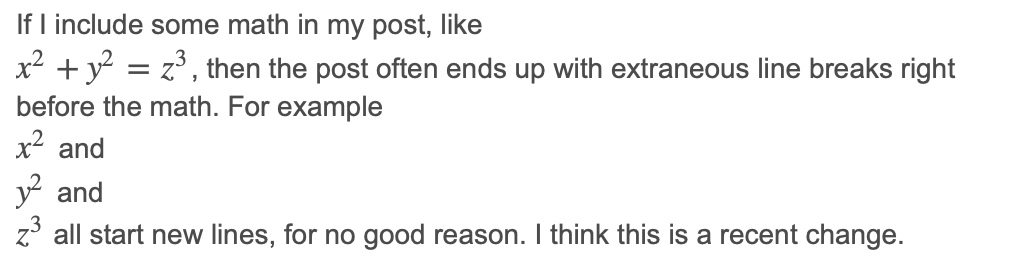# Math typesetting partly broken on SafariIf I include some math, like $x^2 + y^2 = z^3$, in an Ask Sage question or answer, then the post often ends up with an extraneous line break right before each inline math formula, when viewed in Safari on macOS.

For example $x^2$ and $y^2$ and $z^3$ all start new lines, for no good reason. I think this is a recent change.

Here is an earlier version of this same question:

If I include some math in my post, like $x^2 + y^2 = z^3$,
then the post often ends up with extraneous line breaks
right before the math. For example $x^2$ and $y^2$
and $z^3$ all start new lines, for no good reason.
I think this is a recent change.


and a screenshot of how it was rendered in Safari:A web search for [ mathjax safari newline ] reveals similar questions:

The MathJax issue link suggests that it's been fixed in a more recent MathJax version. Can we update the version of MathJax used on this site?

edit retag close merge delete